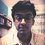# A Calculus Problem!

The Problem:

Find $I=\displaystyle\int_{0}^{2} (x^2+1)\,d\left \lfloor x\right \rfloor$

My Doubt:

There seem two probable approaches to the question but both of them yield different results. Both seem to be mathematically correct and hence the confusion.

Approach 1:

We know that,

$g(x). f'(x) + f(x). g'(x) = \large \frac{d\left(f(x). g(x)\right)}{dx}$

Integrating the above expression we get:

$\displaystyle\int_{a}^{b} g(x)\,df(x) + \displaystyle\int_{a}^{b} f(x)\,dg(x) = f(b).g(b)-f(a).g(a)$

Using the above property to solve the integration we get the answer as $\boxed{7}$

Approach 2:

We can write

$I=\displaystyle\int_{0}^{1} (x^2+1)\,d\left \lfloor x\right \rfloor +\displaystyle\int_{1}^{2}(x^2+1)\,d\left \lfloor x\right \rfloor$

But $\left \lfloor x\right \rfloor$ assumes constant values of $\left \lfloor x\right \rfloor=0$ and $\left \lfloor x\right \rfloor=1$ in the respective intervals. And hence in both cases $d\left \lfloor x\right \rfloor=0$ and therefore $I=0$

I genuinely can't understand what's the correct method. I will really be grateful if someone can explain it to me.

Thank You!Note by Miraj Shah
4 years, 2 months ago

This discussion board is a place to discuss our Daily Challenges and the math and science related to those challenges. Explanations are more than just a solution — they should explain the steps and thinking strategies that you used to obtain the solution. Comments should further the discussion of math and science.

When posting on Brilliant:

• Use the emojis to react to an explanation, whether you're congratulating a job well done , or just really confused .
• Ask specific questions about the challenge or the steps in somebody's explanation. Well-posed questions can add a lot to the discussion, but posting "I don't understand!" doesn't help anyone.
• Try to contribute something new to the discussion, whether it is an extension, generalization or other idea related to the challenge.

MarkdownAppears as
*italics* or _italics_ italics
**bold** or __bold__ bold
- bulleted- list
• bulleted
• list
1. numbered2. list
1. numbered
2. list
Note: you must add a full line of space before and after lists for them to show up correctly
paragraph 1paragraph 2

paragraph 1

paragraph 2

[example link](https://brilliant.org)example link
> This is a quote
This is a quote
    # I indented these lines
# 4 spaces, and now they show
# up as a code block.

print "hello world"
# I indented these lines
# 4 spaces, and now they show
# up as a code block.

print "hello world"
MathAppears as
Remember to wrap math in $$ ... $$ or $ ... $ to ensure proper formatting.
2 \times 3 $2 \times 3$
2^{34} $2^{34}$
a_{i-1} $a_{i-1}$
\frac{2}{3} $\frac{2}{3}$
\sqrt{2} $\sqrt{2}$
\sum_{i=1}^3 $\sum_{i=1}^3$
\sin \theta $\sin \theta$
\boxed{123} $\boxed{123}$

Sort by:

In Approach 2, the floor function fails to be constant on the two subintervals; consider the two endpoints! The first integral takes the value of 1 due to the step at 1, and the second one takes a value of 5. With this correction, both approaches work and yield the same result.

- 4 years, 2 months ago

First off, integration by parts doesn't work as the step function isn't differentiable.

Secondly, the integral is 2. I'll provide a proof of this later.

Btw, @Aditya Kumar The integral makes perfect sense and is defined.

- 4 years, 2 months ago

It would be really nice of you to put the proof here if possible! But I would also like to know then what's wrong with the Approach 1&2 illustrated above? It's a bit baffling

- 4 years, 2 months ago

Wait, the integral is 7.

We shall use the definition of integration as given in this book, with $\alpha(x)=\lfloor x \rfloor$ and $f(x)=1+x^2$.

Consider a partition $P$ of $[0,2]$ such that $x_{r-1}\leq 1\leq x_{r}$ where strict inequality holds in atleast one of the two inequalities. Also, by the definition of a partition, we have $x_{n-1}<2=x_n$. Then,

$U(P, f, \alpha)=f(x_r)+f(x_n) \\ L(P, f, \alpha)=f(x_{r-1})+f(x_{n-1}) \\\implies \inf U(P, f, \alpha)=\sup L(P, f, \alpha)=f(1)+f(2) \\\implies \int_0^2 f \, d\alpha = 7$

Note: I've only used the fact that $f$ is monotonic.

Plus, in the book mentioned above, in the exercises, a more generalised version of IBP, valid for even discontinuos functions is given. You've made use of this version in approach 1 (luckily, the step function is monotonic).

If the integrals are calculated using the definition in approach 2, you get the right answer, 7.

- 4 years, 2 months ago

- 4 years, 2 months ago

Oh yes! Really helpful @Deeparaj Bhat ! Sorry for replying a bit late. Busy studying for the big one which is to be held on 22nd May! Actually the above problem was from a test paper it self! Had a doubt in the question, so thought of taking help from the brilliant Brilliant Community as I was pretty sure that I will be able to get at least some insight!

Thank you!

- 4 years, 2 months ago

You're writing any other exams? (Except JEE ADVANCED, which is on 22nd)

- 4 years, 2 months ago

Actually yes. BITS and all! You have to give JEE this year?

- 4 years, 2 months ago

Yup. That, BITSAT and CMI in my case.

- 4 years, 2 months ago